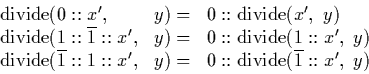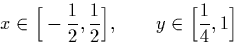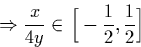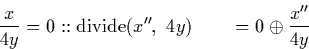Next: Output positive digits Up: Division Previous: Modification of mantissas

#### Output zero case:

If the numerator input starts with a zero, or two digits which could be rewritten as a zero and another digit, the algorithm is simple.The proof is as follows. Here, one can say:Hence we can output a zero. Now to express the remainder of the result as a recursive call, we want to find a stream x'' such thatThis is achieved by setting (x'' = 2x). We can represent 2x using a signed binary stream as the initial two digits of x scanned indicate that it be expressed in the form (0::x').

Martin Escardo
5/11/2000